# 978-0123869449 Chapter 1 Solution Manual Part 2

Document Type
Homework Help
Book Title
Authors
Michael F. Modest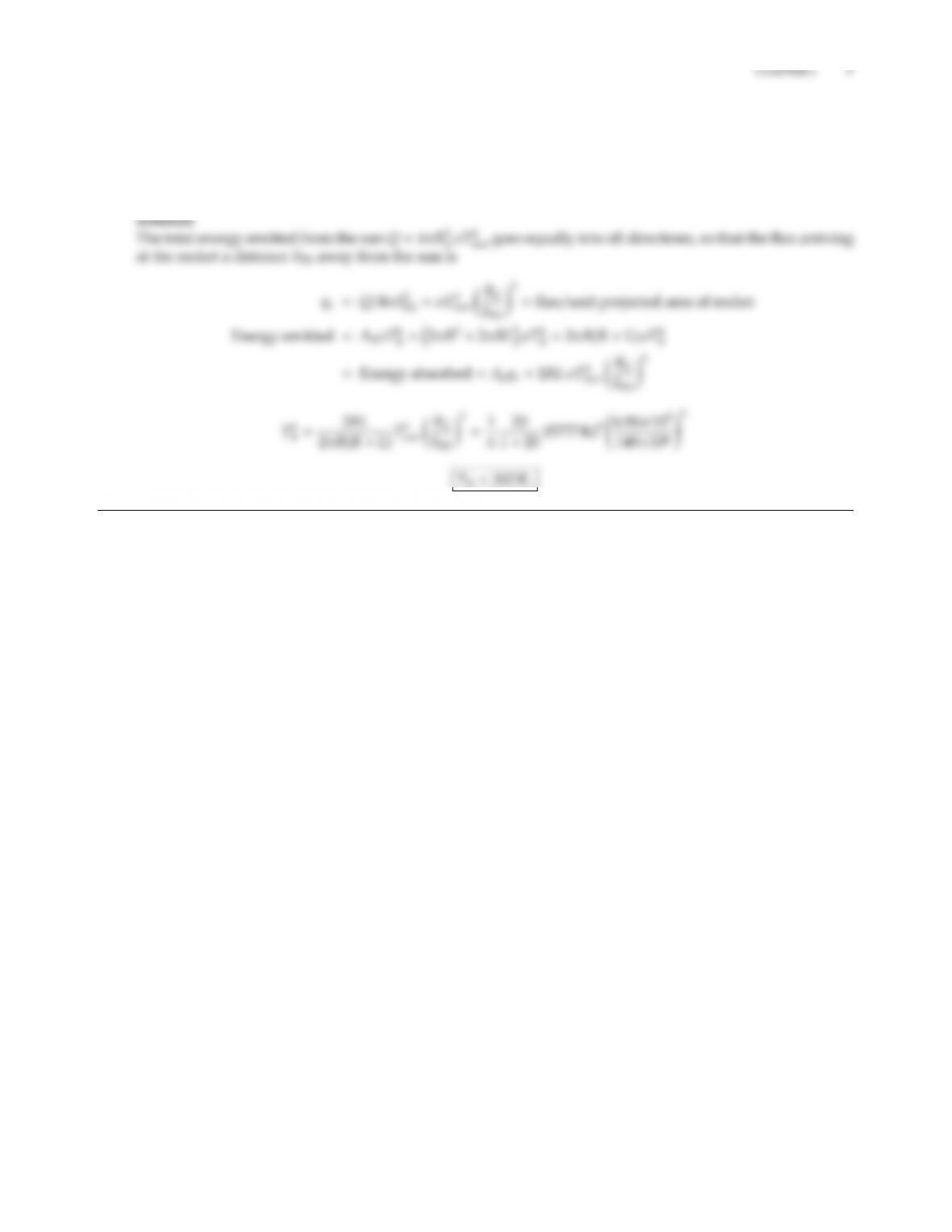1.6 A rocket in space may be approximated as a black cylinder of length L=20 m and diameter D=2 m. It
ﬂies past the sun at a distance of 140 million km such that the cylinder axis is perpendicular to the sun’s
rays. Assuming that (i) the sun is a blackbody at 5777 K and (ii) the cylinder has a high conductivity (i.e.,
is essentially isothermal), what is the temperature of the rocket? (Radius of sun RS=696,000 km; neglect
radiation from earth and the stars).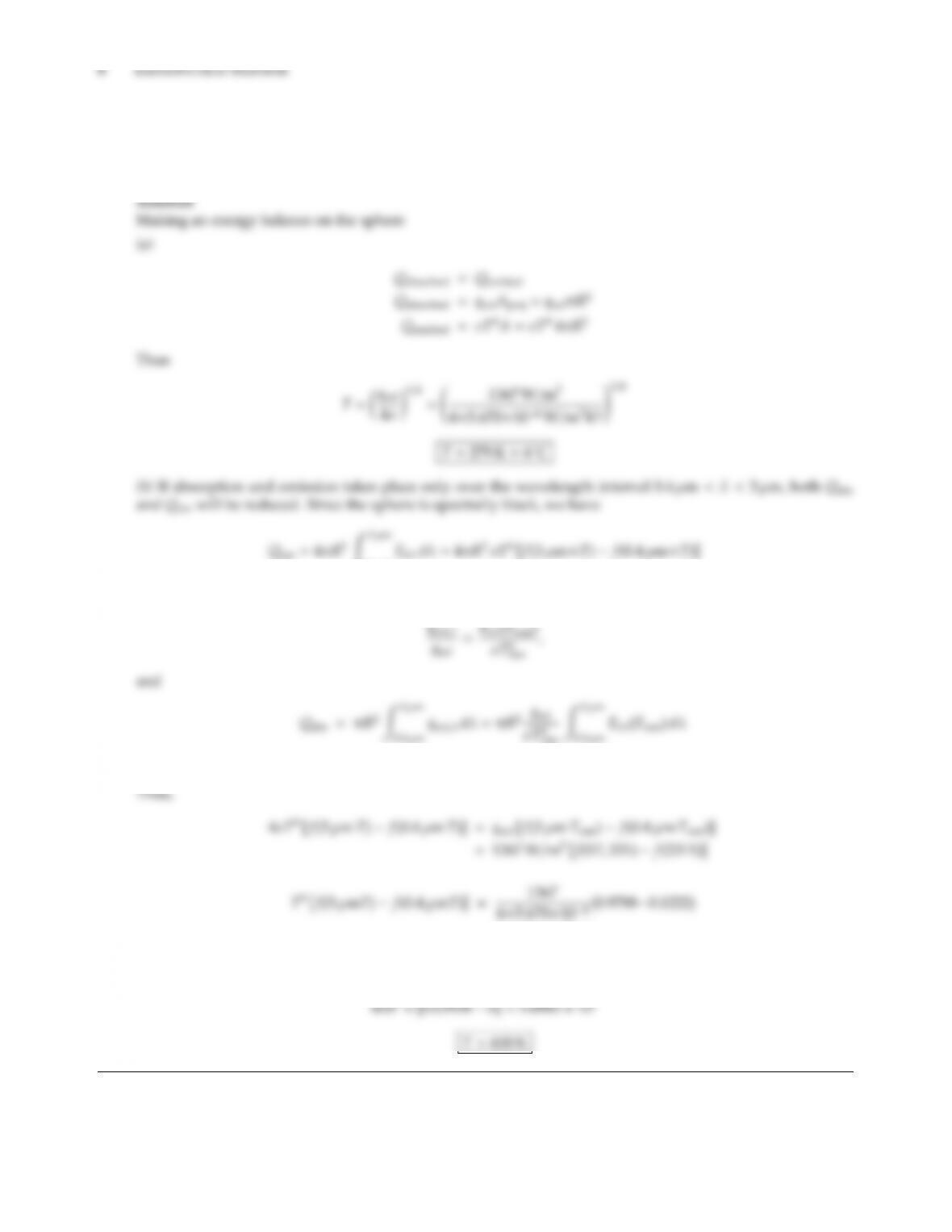1.7 A black sphere of very high conductivity (i.e., isothermal) is orbiting earth. What is its temperature? (Consider
the sun but neglect radiation from the earth and the stars). What would be the temperature of the sphere if
it were coated with a material that behaves like a black body for wavelengths between 0.4µm and 3 µm, but
does not absorb and emit at other wavelengths?
0.4µm
Solar emission is black, i.e., qsol Ebλ(Tsun), or
=πR2qsol f(3 µm×Tsun)f(0.4µm×Tsun)
=5.1630 ×109K4
This nonlinear relation must be solved by iteration, leading to T600 K (<601 K)1.8 A 100 Watt light bulb may be considered to be an isothermal black sphere at a certain temperature. If the
light ﬂux (i.e., visible light, 0.4µm<λ<0.7µm) impinging on the ﬂoor directly (2.5 m) below the bulb is
42.6 mW/m2, what is the light bulb’s eective temperature? What is its eciency?
h=2.5 m)
qﬂoor,visible =qfv =f(λ2T)f(λ1T)Qbulb
=0.03346
By trial and error:
T=2500 K λ1T=0.4µm×2500 K =1000 µm K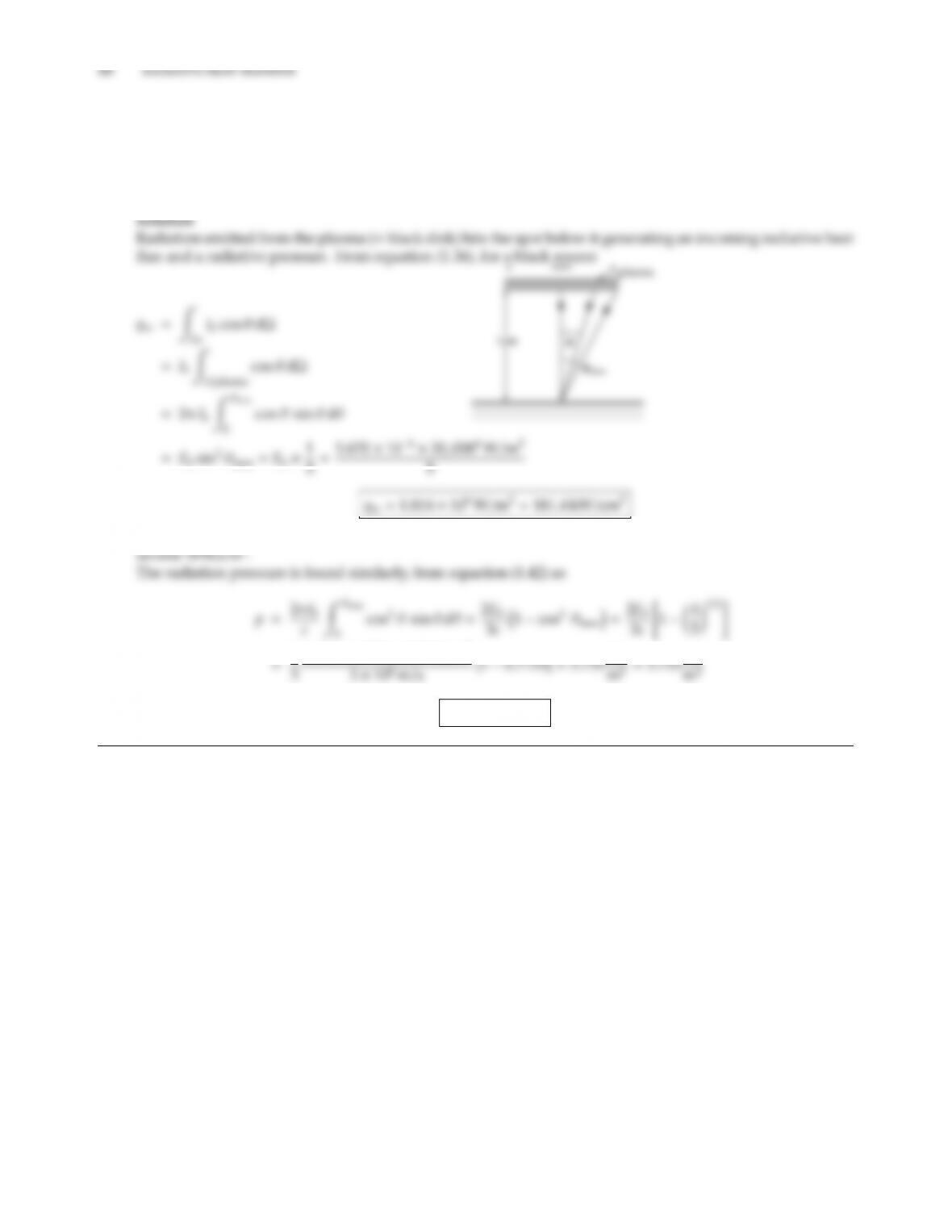1.9 When a metallic surface is irradiated with a highly concentrated laser beam, a plume of plasma (i.e., a gas
consisting of ions and free electrons) is formed above the surface that absorbs the laser’s energy, often blocking
it from reaching the surface. Assume that a plasma of 1 cm diameter is located 1 cm above the surface, and
that the plasma behaves like a blackbody at 20,000 K. Based on these assumptions calculate the radiative heat
ﬂux and the total radiation pressure on the metal directly under the center of the plasma.
This should be compared to the maximum ﬂux at the center of an unobstructed laser beam, which may be
5.670×10820,0004W/m2
p=5.735 N/m2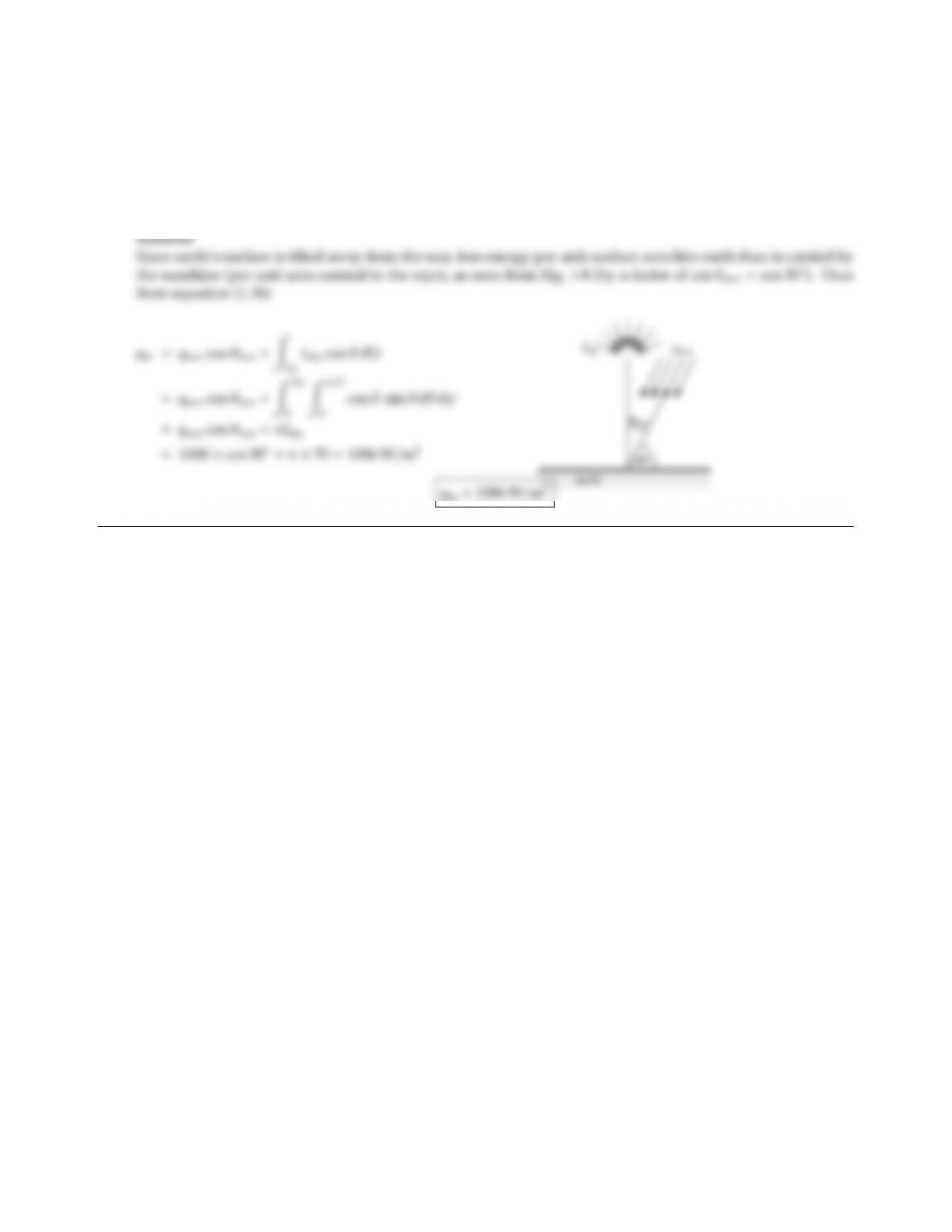CHAPTER 1 11
1.10 Solar energy incident on the surface of the earth may be broken into two parts: A direct component (traveling
unimpeded through the atmosphere) and a sky component (reaching the surface after being scattered by the
atmosphere). On a clear day the direct solar heat ﬂux has been determined as qsun =1000 W/m2(per unit area
normal to the rays), while the intensity of the sky component has been found to be diuse (i.e., the intensity of
the sky radiation hitting the surface is the same for all directions) and Isky =70 W/m2sr. Determine the total
solar irradiation onto earth’s surface if the sun is located 60above the horizon (i.e., 30from the normal).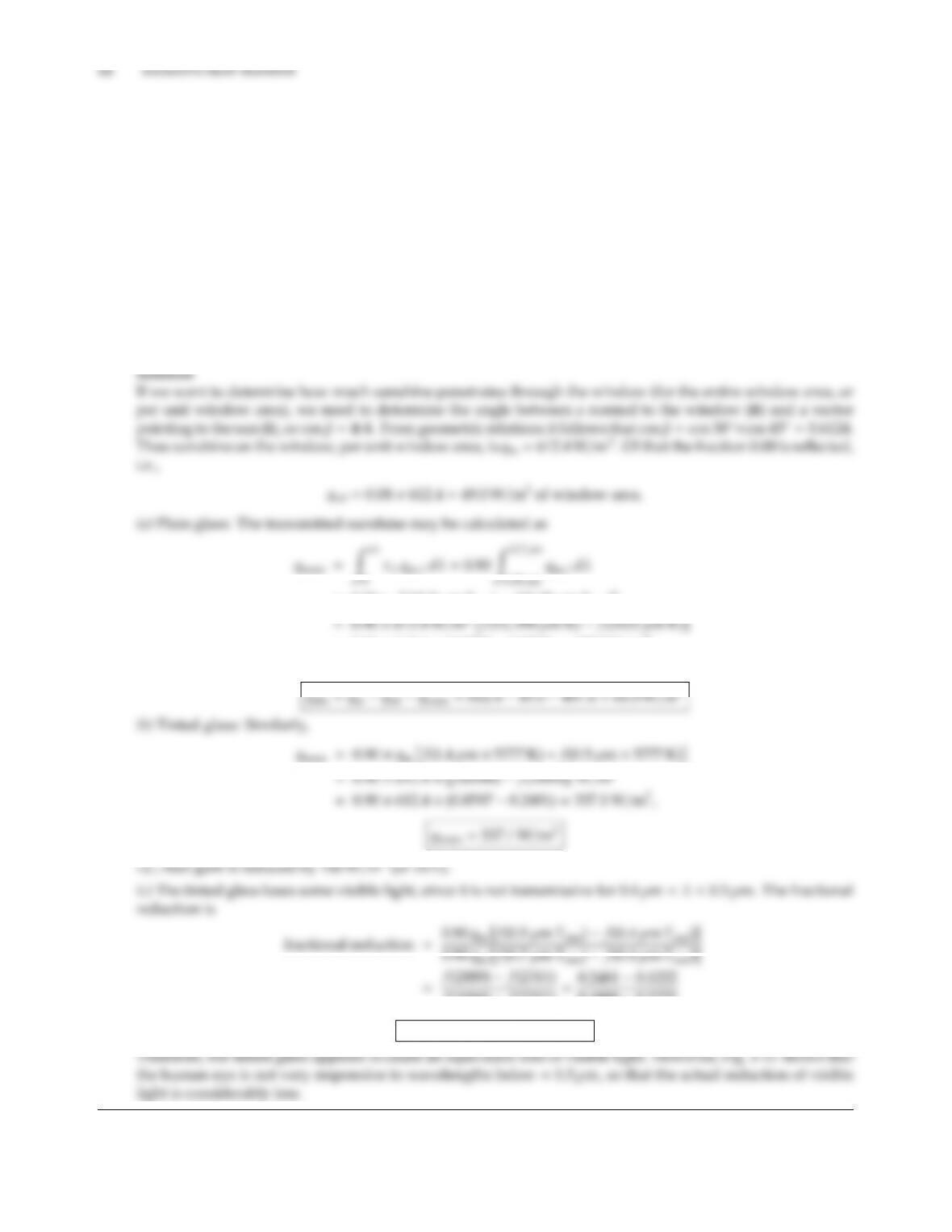1.11 A window (consisting of a vertical sheet of glass) is exposed to direct sunshine at a strength of 1000 W/m2.
The window is pointing due south, while the sun is in the southwest, 30above the horizon. Estimate the
amount of solar energy that (i) penetrates into the building, (ii) is absorbed by the window, and (iii) is reﬂected
by the window. The window is made of (a) plain glass, (b) tinted glass, whose radiative properties may be
approximated by
ρλ=0.08 for all wavelengths (both glasses),
τλ=0.90 for 0.35 µm<λ<2.7µm
0 for all other wavelengths (plain glass)
τλ=0.90 for 0.5µm<λ<1.4µm
0 for all other wavelengths (tinted glass).
(c) By what fraction is the amount of visible light (0.4µm< λ < 0.7µm) reduced, if tinted rather that plain
glass is used? How would you modify this statement in the light of Fig. 1-11?
=0.90 qin f(2.7µmTsun)f(0.35 µmTsun)
=0.90 ×612.4×(0.9720 0.0702) =497.0 W/m2
It follows that
f(4044) f(2311) =0.2481 0.1222
0.4888 0.1222
fractional reduction =34.3%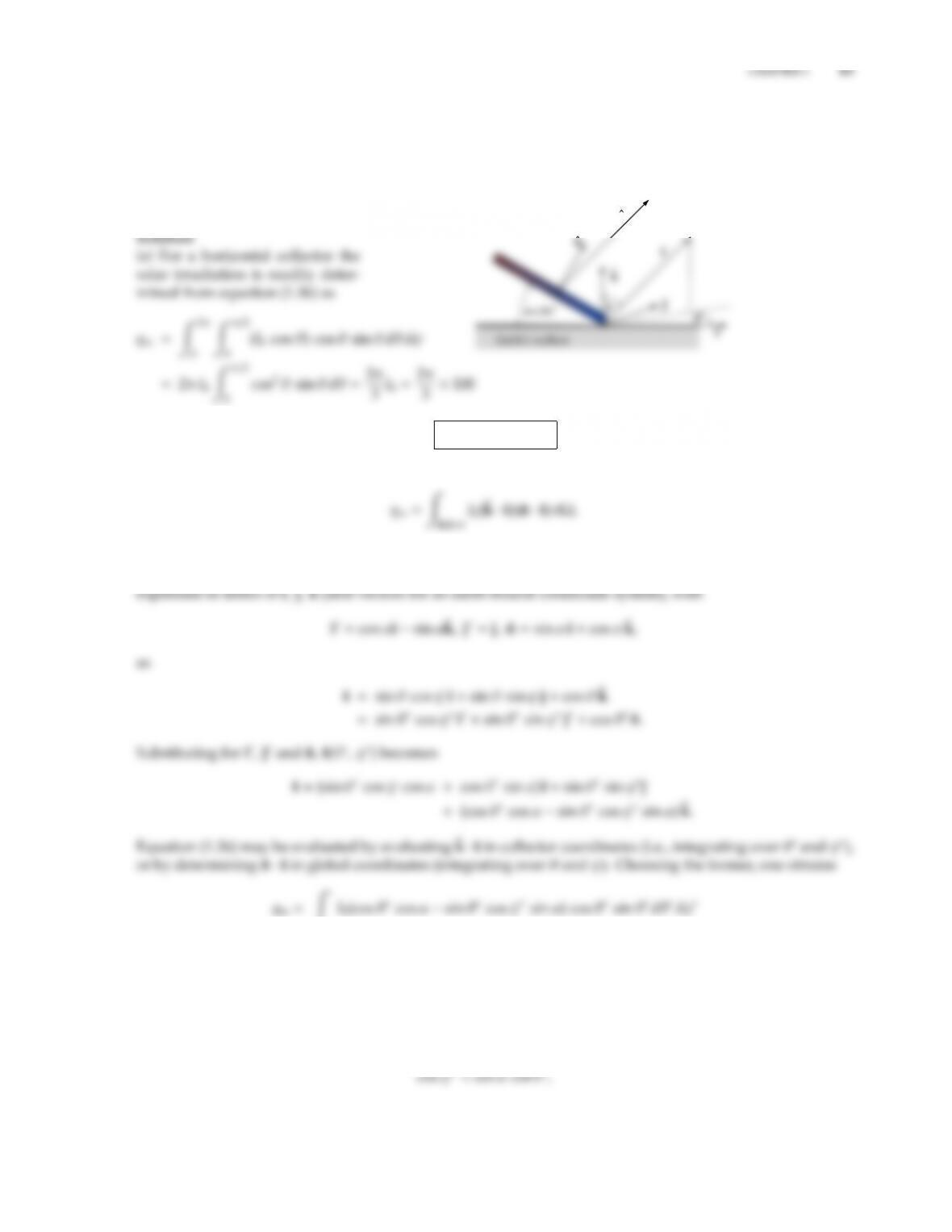1.12 On an overcast day the directional behavior of the intensity of solar radiation reaching the surface of the
earth after being scattered by the atmosphere may be approximated as Isky(θ)=Isky(θ=0) cos θ, where θ
is measured from the surface normal. For a day with Isky(0) =100 W/m2sr determine the solar irradiation
hitting a solar collector, if the collector is (a) horizontal, (b) tilted from the horizontal by 30. Neglect radiation
from the earth’s surface hitting the collector (by emission or reﬂection).
s
qin =209.4 W/m2
(b) To evaluate qin for a tilted collector, equation (1.36) becomes
where ˆ
k·ˆs =cos θgives the variation of sky intensity with sky polar angle θ, while ˆn ·ˆs =cos θis the cosine
of the angle between the surface normal and a unit vector pointing into the sky, ˆs. This unit vector may be
ˆ
k·ˆs>0
ˆn·ˆs>0
The integration limits imply that only directions above the collector (0 < θ < π/2) and above the horizontal
ˆ
k·ˆs =cos θ=cos θcos αsin θcos ψsin α > 0
are to be considered. Solving the last expression for cos ψyields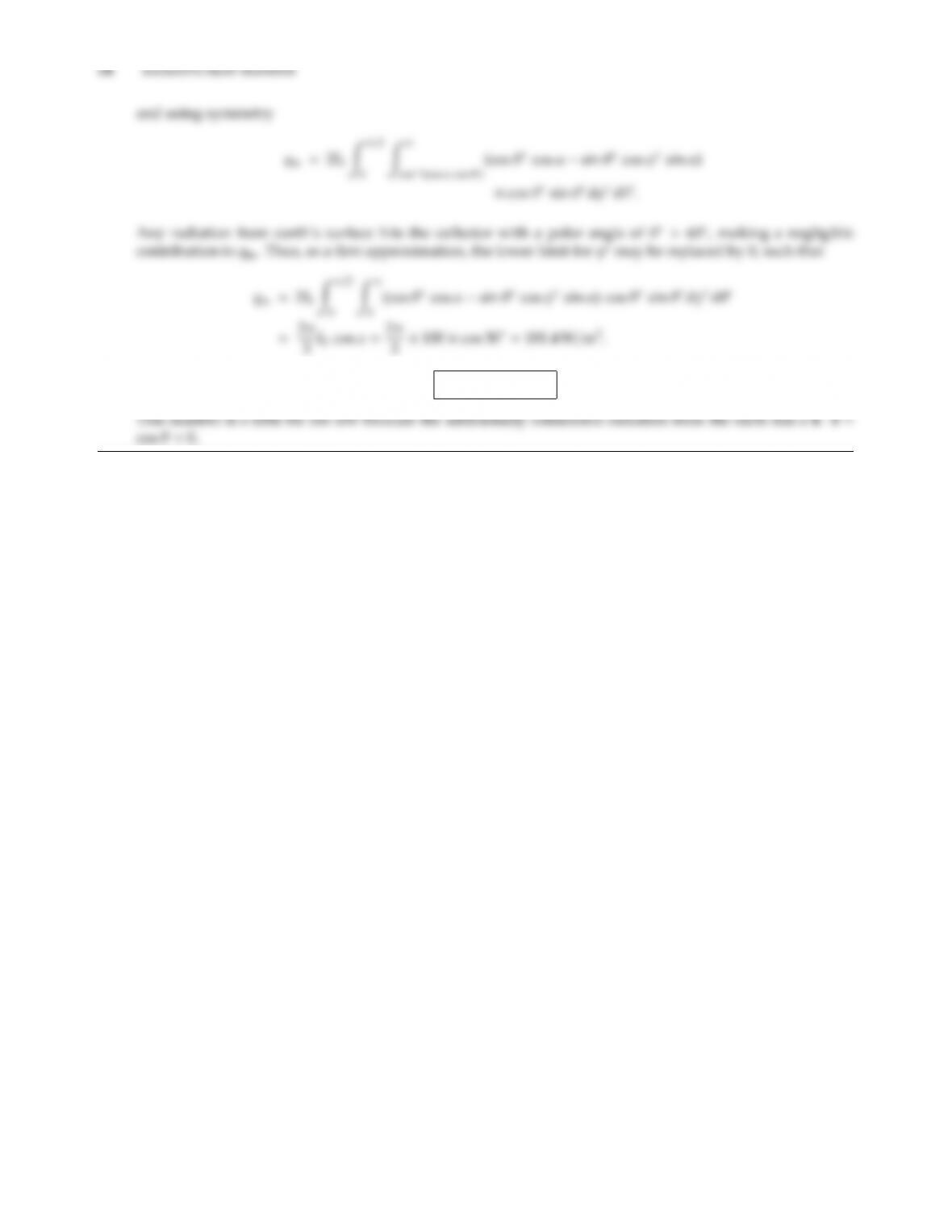qin =181.4 W/m2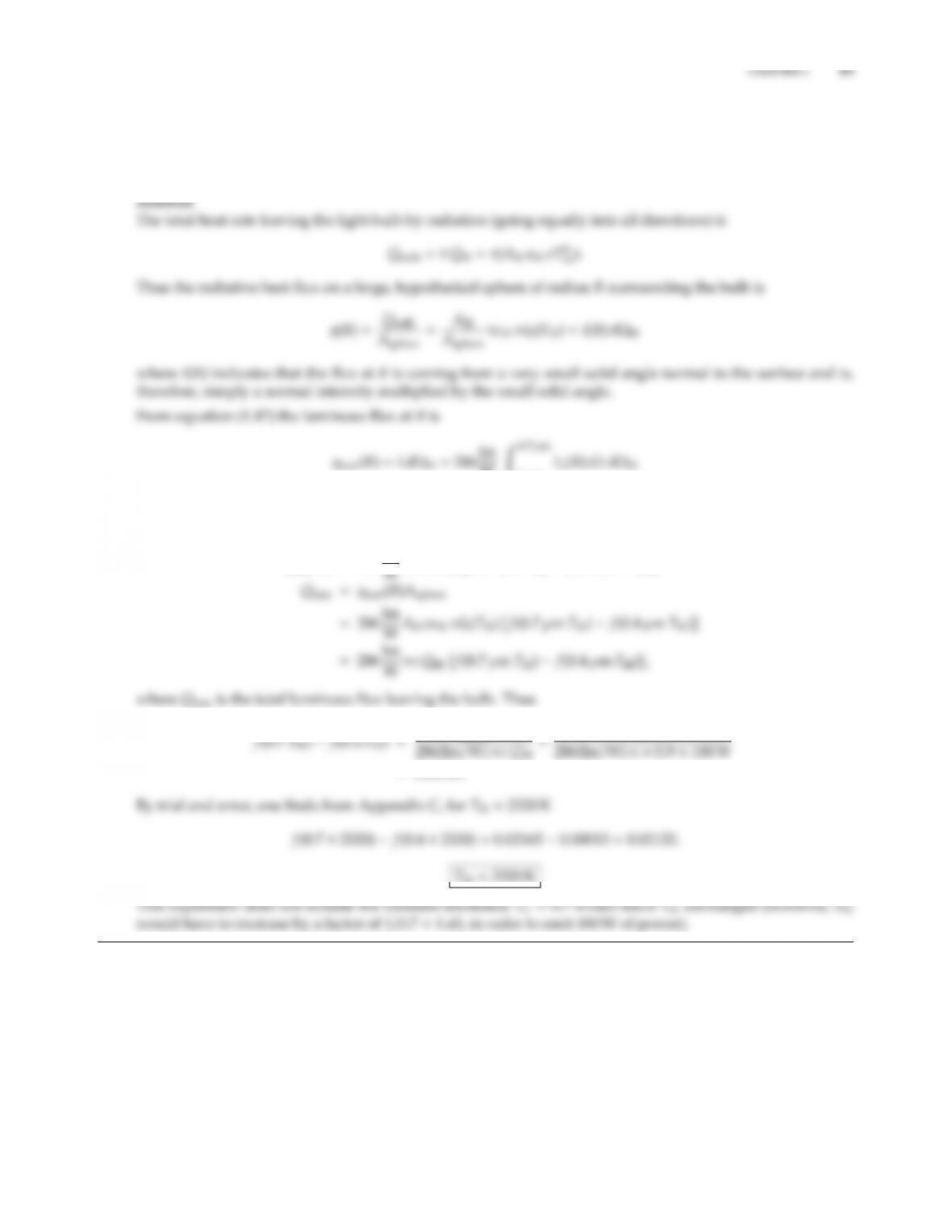1.13 A 100 W light bulb is rated to have a total light output of 1750 lm. Assuming the light bulb to consist of a
small, black, radiating body (the light filament) enclosed in a glass envelope (with a transmittance τ1=0.9
throughout the visible wavelengths), estimate the filament’s temperature. If the filament has an emittance of
ǫf=0.7 (constant for all wavelengths and directions), how does it aect its temperature?
WZ0.7µm
0.4µm
With gray (i.e., constant) values for ǫfil and τ(at least over the visible wavelengths), this makes Iλ(R)Ibλ(Tfil),
and
qlum(R)=286lm
=0.02164.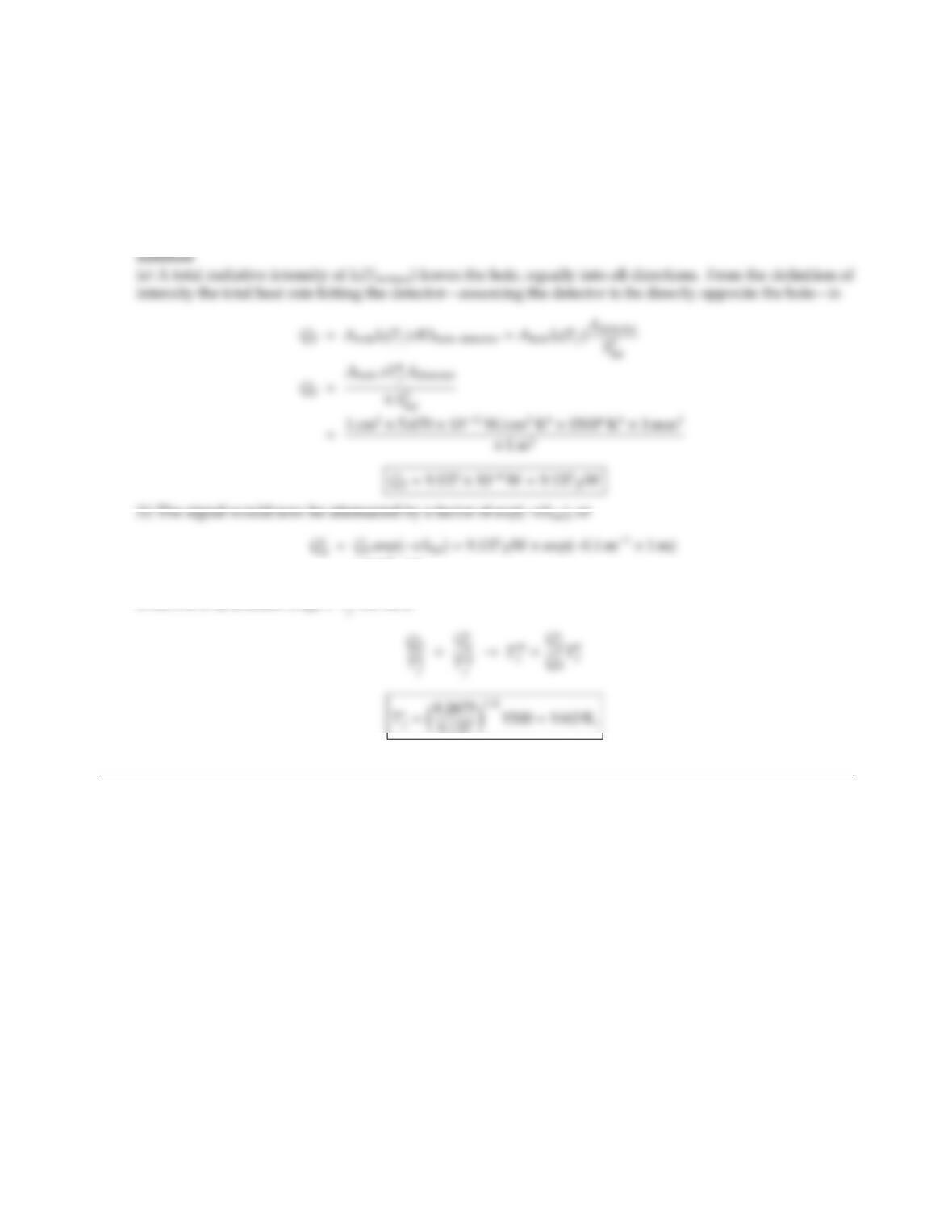1.14 Apyrometer is a device with which the temperature of a surface may be determined remotely by measuring
the radiative energy falling onto a detector. Consider a black detector of 1 mm ×1 mm area, that is exposed
to a 1 cm2hole in a furnace located a distance of 1 m away. The inside of the furnace is at 1500 K and
the intensity escaping from the hole is essentially blackbody intensity at that temperature. (a) What is the
radiative heat rate hitting the detector? (b) Assuming that the pyrometer has been calibrated for the situation
in (a), what temperature would the pyrometer indicate if the nonabsorbing gas between furnace and detector
were replaced by one with an (average) absorption coecient of κ=0.1 m1?
=8.2675 µW
Since the d calibration is QdT4
9.137 1/4
i.e., the measurement would be wrong by 37 K.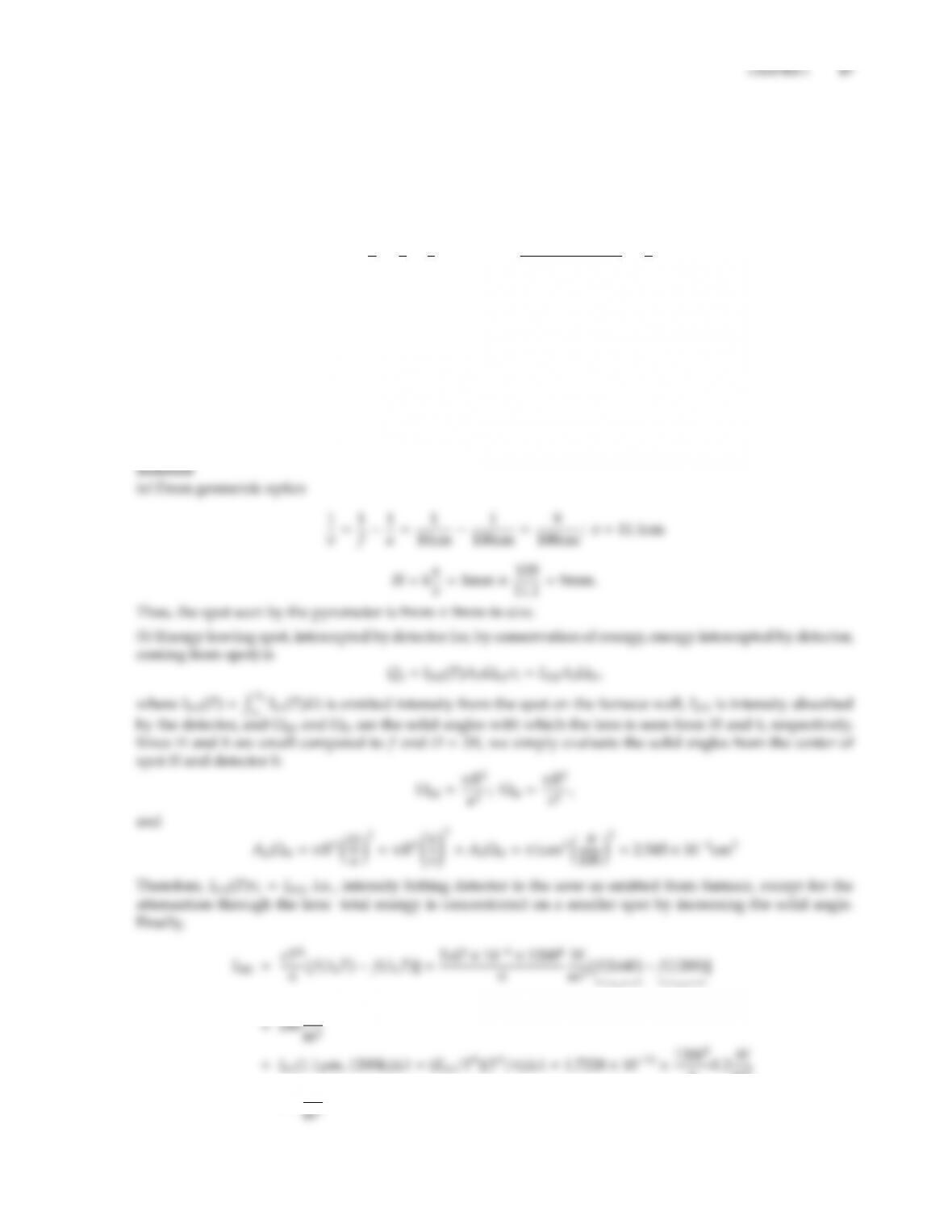1.15 Consider a pyrometer, which also has a detector area of 1mm ×1mm, which is black in the wavelength range
1.0µmλ1.2µm, and perfectly reﬂecting elsewhere. In front of the detector is a focussing lens ( f=10 cm)
of diameter D=2 cm, and transmissivity of τl=0.9 (around 1µm). In order to measure the temperature
inside a furnace, the pyrometer is focussed onto a hot black surface inside the furnace, a distance of 1 m away
from the lens.
(a) How large a spot on the furnace wall does the detector see? (Remember that geometric optics dictates
1
f=1
u+1
v;M=h(detector size)
Hspot size=v
u,
where u=1m is the distance from lens to furnace wall, and vis the distance from lens to detector.)
(b) If the temperature of the furnace wall is 1200 K, how much energy is absorbed by the detector per unit
time?
(c) It turns out the furnace wall is not really black, but has an emittance of ǫ=0.7 (around 1µm). Assuming
there is no radiation reﬂected from the furnace surface reaching the detector, what is the true surface
temperature for the pyrometer reading of case (b)?
(d) To measure higher temperatures pyrometers are outfitted with filters. If a τf=0.7 filter is placed in front
of the lens, what furnace temperature would provide the same pyrometer reading as case (b)?
0.00961
0.00213
π0.2W
m2
=273 W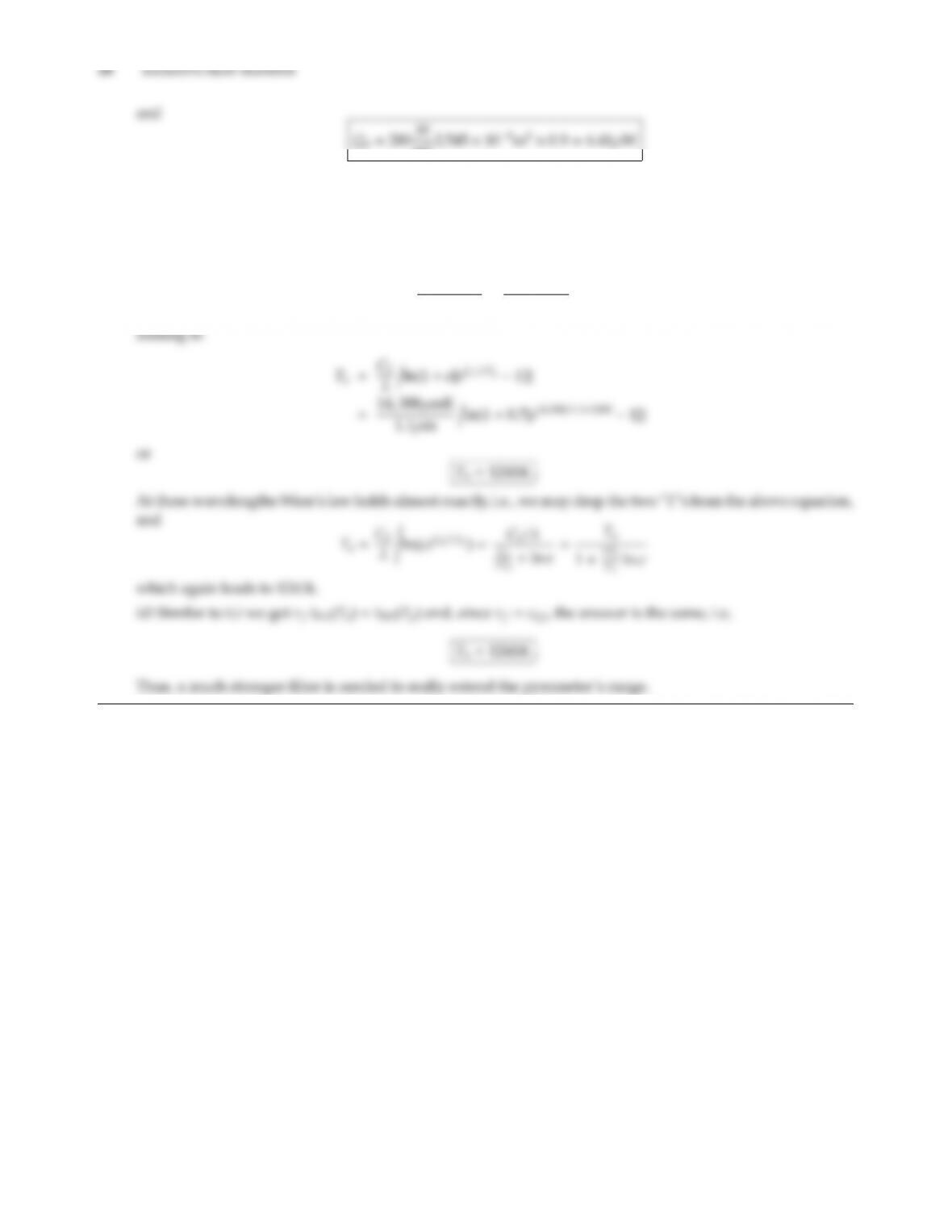m22.545 ×108m2×0.9=6.41µW
(c) The energy hitting detector remains the same and, therefore, so does the intensity emitted from the spot:
ǫIb12(Ta)(actual) =Ib12(Tp=1200K)(perceived)
or, if we assume the blackbody intensity over the detector range can be approximated by the value at 1.1µm,
ǫ
eC2Ta11
eC2Tp1,

## Trusted by Thousands ofStudents

Here are what students say about us.